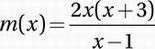# SAT Math Multiple Choice Question 609: Answer and Explanation

### Test Information

Question: 609

9. If the function m(x) satisfies the equation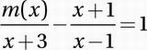for all values of x greater than 1, then m(x) =

• A.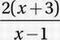• B.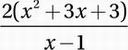• C.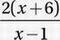• D.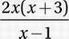Explanation:

D Advanced Mathematics (rational equations) MEDIUM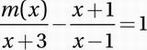Add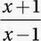: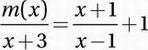Express right side in terms of a common denominator: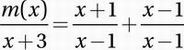Combine terms on right into one fraction: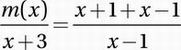Combine terms: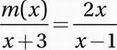Multiple by x + 3: